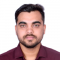## Boxplot stratified by column in Python PandasRishikesh Kumar Rishi

Updated on 02-Feb-2022 10:06:50

To create a boxplot stratified by column in Python class, we can take the following steps −StepsSet the figure size and adjust the padding between and around the subplots.Create a Pandas data frame of two-dimensional, size-mutable, potentially heterogeneous tabular data.Compute the histogram of a set of data.Create a boxplot startified ... Read More

## How to plot aggregated by date pandas dataframe?Rishikesh Kumar Rishi

Updated on 02-Feb-2022 10:01:27

To plot aggregated by date pandas dataframe, we can take the following steps −StepsSet the figure size and adjust the padding between and around the subplots.Create a data frame, df, of two-dimensional, size-mutable, potentially heterogeneous tabular data.Get the values of aggregated by date pandas dataframe.Plot the df (Step 3) with ... Read More

## How to vary the line color with data index for line graph in matplotlib?Rishikesh Kumar Rishi

Updated on 02-Feb-2022 09:49:55

To have the line color vary with the data index for a line graph in matplotlib, we can take the following steps −StepsSet the figure size and adjust the padding between and around the subplots.Create x and y data points using numpy.Get smaller limit, dydx.Get the points and segments data ... Read More

## How can I format a float using matplotlib's LaTeX formatter?Rishikesh Kumar Rishi

Updated on 01-Feb-2022 12:18:00

To format a float using matplotlib's LaTeX formatter, we can take the following steps −StepsSet the figure size and adjust the padding between and around the subplots.Create x and y data points using numpy.Plot the x and y data points using plot() method.Fill the area between the curve.Set the title ... Read More

## How to set local rcParams or rcParams for one figure in matplotlib?Rishikesh Kumar Rishi

Updated on 01-Feb-2022 12:14:03

To set local rcParams or rcParams for one figure in matplotlib, we can take the following steps −StepsSet the figure size and adjust the padding between and around the subplots.Initialize a variable N to store the number of sample data.Create x and y data points using numpy.Return a context manager ... Read More

## Plot multiple boxplots in one graph in Pandas or MatplotlibRishikesh Kumar Rishi

Updated on 01-Feb-2022 12:11:17

To plot multiple boxplots in one graph in Pandas or Matplotlib, we can take the following steps −StepsSet the figure size and adjust the padding between and around the subplots.Make a Pandas data frame with two columns.Plot the data frame using plot() method, with kind='boxplot'.To display the figure, use show() ... Read More

## How to plot a multivariate function in Python Matplotlib?Rishikesh Kumar Rishi

Updated on 01-Feb-2022 12:05:11

To plot a multivariate function in Python, we can take the following steps −StepsSet the figure size and adjust the padding between and around the subplots.Create random x, y and z data points using numpy.Create a figure and a set of subplots.Create a scatter plot with x, y and z ... Read More

## How to make a polygon radar (spider) chart in Python Matplotlib?Rishikesh Kumar Rishi

Updated on 01-Feb-2022 11:57:55

To make a polygon radar (spider) chart in Python, we can take the following steps −StepsSet the figure size and adjust the padding between and around the subplots.Create a Pandas dataframe with sports and values columns.Create a new figure or activate an existing figure.Add an 'ax' to the figure as ... Read More

## Add a legend in a 3D scatterplot with scatter() in MatplotlibRishikesh Kumar Rishi

Updated on 01-Feb-2022 11:52:09

To add a legend in a 3D scatterplot with scatter() in matplotlib, we can take the following steps −StepsSet the figure size and adjust the padding between and around the subplots.Initialize a variable N to store the number of sample data.Create x and y data points; make z1 and z2 ... Read More

## Remove NaN values from a dataframe without fillna or Interpolate (Python Matplotlib)Rishikesh Kumar Rishi

Updated on 01-Feb-2022 11:47:54

To remove NaN values from a dataframe without filter or interpolate, we can take the following steps −StepsSet the figure size and adjust the padding between and around the subplots.Create an array to make a Pandas data frame.One-dimensional ndarray with axis labels (including time series).Plotting interpolation, 'index', 'values' − Use ... Read More